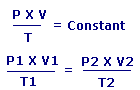Combined Gas Law CalculatorP1= Pressure 1 V1= Volume 1 T1= Temperature 1 P2= Pressure 2 V2= Volume 2 T2= Temperature 2

Click on Calculate Pressure or Calculate Volume or Calculate Temperature you wish to calculate.

or or

Enter value and click on calculate. Result will be displayed.

 Enter your values: : : : : :
 Results: :

 Web www.calculatoredge.com

The Combined Gas Law is a gas law which combines Charles's law, Boyle's law, and Gay-Lussac's law.

Charles's law states that volume and temperature are directly proportional to each other while pressure is held constant. Boyle's law asserts that pressure and volume are inversely proportional to each other at fixed temperature. Finally Gay-Lussac's law introduces a direct proportionality between temperature and pressure at constant volume. The inter-dependence of these variables is shown in the combined gas law.

Combined Gas Law states that: The ratio between the Pressure-volume constant and Temperature of a system remains constant.LINKSDISCLAIMERCONTACT US